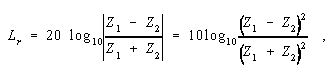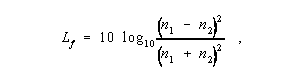Back

## reflection loss

At a discontinuity or impedance mismatch, e.g., in a transmission line, the ratio of the incident power to the reflected power. Note 1: Reflection loss is usually expressed in dB. Note 2: The reflection loss, Lr, is given bywhere Z1 and Z2 are the respective impedances, and the vertical bars designate absolute magnitude. 2. In an optical fiber, the loss that takes place at any discontinuity of refractive index, especially at an air-glass interface such as a fiber endface, at which a fraction of the optical signal is reflected back toward the source. Note: This reflection phenomenon is also called “Fresnel reflection loss,” or simply, “Fresnel loss.”

At normal incidence, the fraction of reflected power is expressed by the formula,where n1 and n2 are the respective indices of refraction.